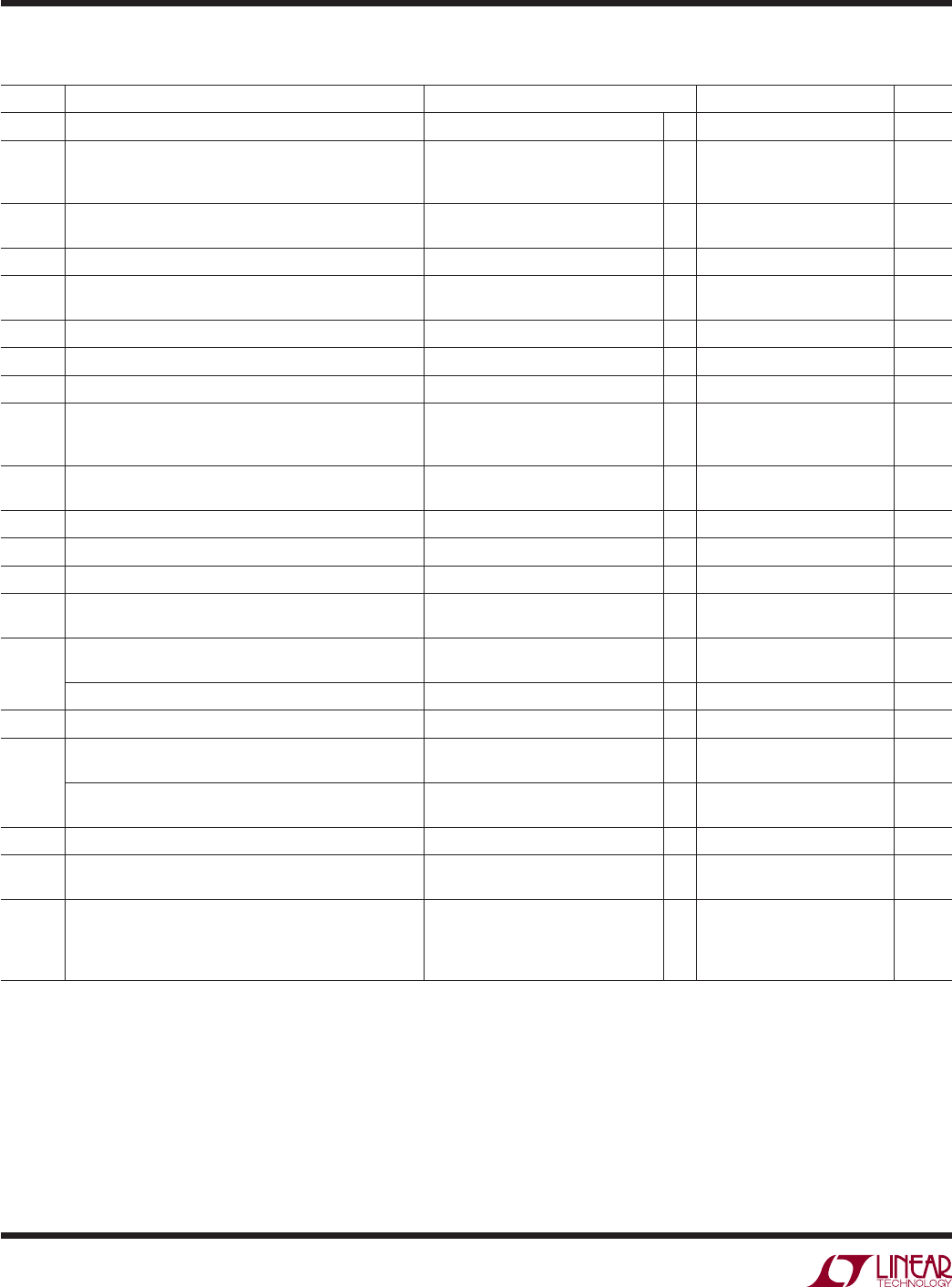# DatasheetLT1785/LT1785A/
LT1791/LT1791A
4
178591fc
DC ELECTRICAL CHARACTERISTICS
The l denotes the speciﬁ cations which apply over the full operating
temperature range, otherwise speciﬁ cations are at T
A
= 25°C, V
CC
= 5V.
SYMBOL PARAMETER CONDITIONS MIN TYP MAX UNITS
V
OD1
Differential Driver Output Voltage (Unloaded) I
O
= 0
l
4.1 5 V
V
OD2
Differential Driver Output Voltage (With Load) R = 50 (RS422), Figure 1
R = 27 (RS485), Figure 1
R = 18
l
l
l
2.0
1.5
1.2
2.70
2.45
2.2
V
V
V
V
OD
Change in Magnitude of Driver Differential Output
Voltage for Complementary Output States
R = 27 or R = 50, Figure 1
l
0.2 V
V
OC
Driver Common Mode Output Voltage R = 27 or R = 50, Figure 1
l
2 2.5 3 V
∆|V
OC
| Change in Magnitude of Driver Common Mode Output
Voltage for Complementary Output States
R = 27 or R = 50, Figure 1
l
0.2 V
V
IH
Input High Voltage DI, DE, RE
l
2V
V
IL
Input Low Voltage DI, DE, RE
l
0.8 V
I
IN1
Input Current DI, DE, RE
l
A
I
IN2
Input Current (A, B); (LT1791 or LT1785 with DE = 0V) V
IN
= 12V
V
IN
= –7V
–60V ≤ V
IN
≤ 60V
l
l
l
–0.15
–6
0.15
–0.08
0.3
6
mA
mA
mA
V
TH
Differential Input Threshold Voltage for Receiver LT1785/LT1791: –7V ≤ V
CM
≤ 12V
LT1785A/LT1791A: –7V ≤ V
CM
≤ 12V
l
l
–0.2
–0.2
0.2
0
V
V
∆V
TH
Receiver Input Hysteresis –7V < V
CM
< 12V 20 mV
V
OH
O
= –400µA, V
ID
= 200mV
l
3.5 4 V
V
OL
O
= 1.6mA, V
ID
= –200mV
l
0.3 0.5 V
Three-State (High Impedance) Output Current at
OUT
< 6V
RE > 2V or Power Off
l
–1 1 µA
R
IN
Receiver Input Resistance (LT1791) –7V ≤ V
CM
≤ 12V
– 60V ≤ V
CM
≤ 60V
l
85 125
125
kΩ
kΩ
LT1785 –7V ≤ V
CM
≤ 12V
l
50 90 kΩ
I
SC
Driver Short-Circuit Current V
OUT
= HIGH, Force V
O
= –7V
V
OUT
= LOW, Force V
O
= 12V
l
l
35
35
250
250
mA
mA
Driver Output Fault Current V
O
= 60V
V
O
= –60V
l
l
–6
6mA
mA
Receiver Short-Circuit Current 0V ≤ V
O
≤ V
CC
l
±35 mA
Driver Three-State Output Current –7V ≤ V
O
≤ 12V
–60V ≤ V
O
≤ 60V
l
l
–0.2
–6
0.3
6
mA
mA
I
CC
Supply Current No Load, RE = 0V, DE = 5V
No Load, RE = 5V, DE = 5V
No Load, RE = 0V, DE = 0V
No Load, RE = 5V, DE = 0V
l
l
l
l
5.5
5.5
4.5
0.2
9
9
8
0.3
mA
mA
mA
mA